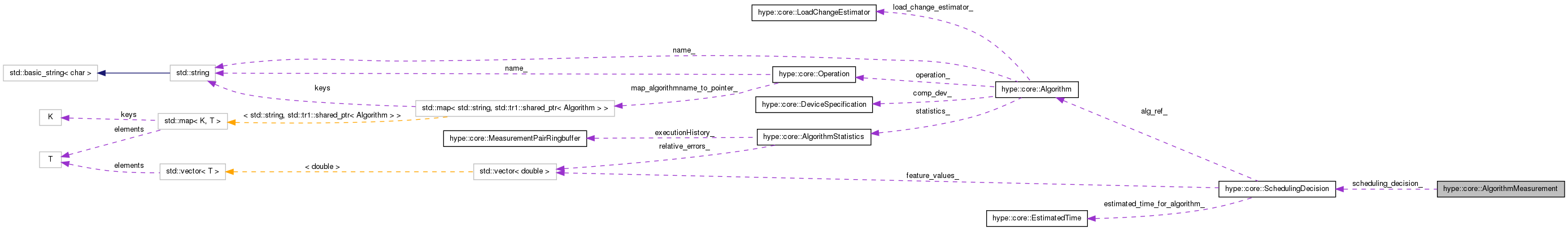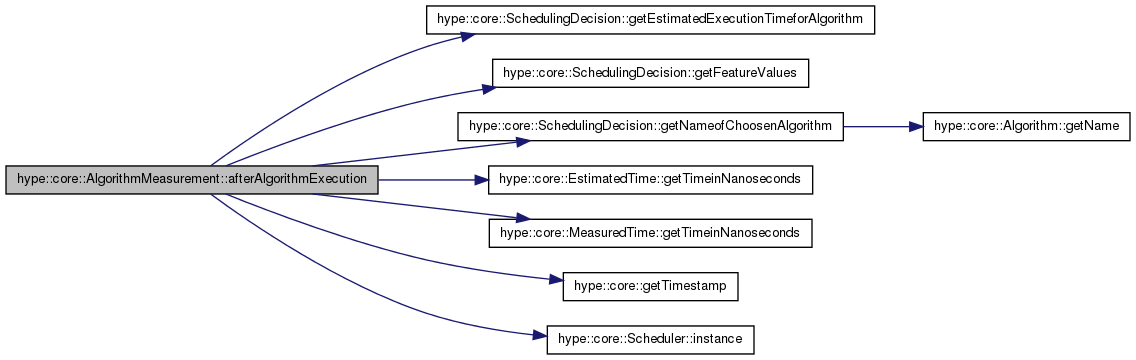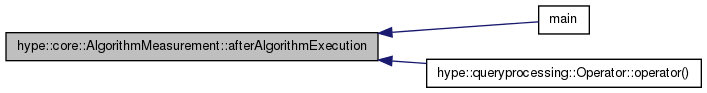Hybrid Query Processing Engine for Coprocessing in Database Systems HyPE
hype::core::AlgorithmMeasurement Class Reference

This class represents an easy to use interface for time measurement of an algorithms execution time. More...

`#include <algorithm_measurement.hpp>`

Collaboration diagram for hype::core::AlgorithmMeasurement:[legend]

List of all members.

## Public Member Functions

AlgorithmMeasurement (const SchedulingDecision &scheduling_decision)
constructs an AlgorithmMeasurement object
void afterAlgorithmExecution ()
stops the time of an algorithms execution and adds obtained data to algorithm statistics.

## Private Attributes

uint64_t timestamp_begin_
timestamp fetched at construction time, used for time measurement
SchedulingDecision scheduling_decision_
scheduling decision, where this object belongs to

## Detailed Description

The user just have to create an object of AlgorithmMeasurement. Then, the algorithm that is to measure is executed. After that, the user has to call afterAlgorithmExecution(). The framework takes care of the rest.

Internally, afterAlgorithmExecution() stops the time and adds the received data to the algorithms statistics. It is crucial that the application executes the algortihm specified by the SchedulingDecision or the libraries behaviour is undefined.

Version:
0.1
Date:
2012
Examples:
online_learning.cpp.

Definition at line 36 of file algorithm_measurement.hpp.

## Constructor & Destructor Documentation

 hype::core::AlgorithmMeasurement::AlgorithmMeasurement ( const SchedulingDecision & scheduling_decision ) ` [explicit]`

The constructor will fetch the current time. Therefore, it starts a timer to measure the execution time of the choosen algorithm. Therefore, the user should construct the AlgorithmMeasurement object directly before the algorithms call. Directly after the algorthm finished execution, afterAlgorithmExecution() has to be called to ensure a precise time measurement.

Parameters:
 cheduling_decision a reference to a SchedulingDecision

Definition at line 12 of file algorithm_measurement.cpp.

## Member Function Documentation

 void hype::core::AlgorithmMeasurement::afterAlgorithmExecution ( )

To ensure a precise time measurement, afterAlgorithmExecution() has to be called directly after the algorthm finished execution.

Definition at line 19 of file algorithm_measurement.cpp.

Referenced by main(), and hype::queryprocessing::Operator::operator()().

Here is the call graph for this function:Here is the caller graph for this function:## Member Data Documentation

 SchedulingDecision hype::core::AlgorithmMeasurement::scheduling_decision_` [private]`

Definition at line 54 of file algorithm_measurement.hpp.

Referenced by afterAlgorithmExecution().

 uint64_t hype::core::AlgorithmMeasurement::timestamp_begin_` [private]`

Definition at line 52 of file algorithm_measurement.hpp.

Referenced by afterAlgorithmExecution().

The documentation for this class was generated from the following files: